# Japanese Mah Jong Scoring

Only the winning hand is evaluated and scored; all other hands are irrelevant.

First, a TALLY (fu [符]) value is calculated based on the composition of the hand and how the hand was won. (I called this term "abscissa" in an earlier version of this draft.) This number will be an integer, usually between 20 and 70.

Then, an EXPONENTIAL (fan [翻]) value is calculated. The significant portion of the exponential (but not all of it) is based on certain properties of the hand which award MULTIPLIERS (yaku [役]). (Since each multiplier will equal one exponential, you'll often see texts confusing the two terms.) The exponential will usually be less than 10.

The TALLY is multiplied by 2^EXPONENTIAL (2 raised to the power of EXPONENTIAL), and is then multiplied by another number depending on whether the winner was dealer or not. Finally, the number is rounded to the nearest LIMIT (kan [貫]) and/or hundred.

If the winning hand is aspecial hand the tally and exponential method is not used.

## Calculating the Tally

The tally is a sum of five numbers; a BASE score, a CLOSURE bonus, a SELF-DRAW bonus, a COMPOSITION score, and a WAITING score. However, there are two exceptions: the SEVEN PAIRS (chiitoitsu [七対子]) hands and the ALL SEQUENCE (pinfu [平和]) hands.

### Base Score (fuudii [副底] or kihonfu [基本符])

The Base score is always 20 points.

### Closure Bonus (menzenron-agari [門前ロンアガり])

If the hand is weakly closed (player won by opponents discard, but has not used any other discards), add 10 points.

### Self-draw Bonus (tsumo-agari [ツモアガり])

If the hand was won by self-draw, add 2 points.

### Composition Score

This score is based on the composition of the hand, regardless of readiness or openness.

The pair is worth:

• 4 points - double (round and position) wind
• 2 points - round wind but not position wind
• 2 points - position wind but not round wind
• 2 points - element;
• no points - guest wind
• no points - suit

Note that the pairs that give points are exactly those SPECIAL CHARS (yakuhai [役牌]) that would give MULTIPLIERS if they were a tri or quad.

Each seq is worth:

• no points.

Each tri is worth:

• 8 points - closed end
• 4 points - open end
• 4 points - closed middle
• 2 points - open middle

• 32 points - closed end
• 16 points - open end
• 16 points - closed middle
• 8 points - open middle

This score is dependent on how exactly the winning tile came into the hand. There are five essential ways:

1. TWO-SIDED (ryanmen [両面]) - the tile completed a seq meld that could have been completed by another number three away from it. For example, holding a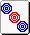and readying for a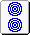or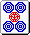.

2. CENTRAL (kanchan [嵌張]) -- the tile completed a seq that was between two numbers. For example, holding a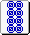and readying for a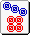.

3. EDGE (penchan [辺張]) - the tile completed a seq that was at the end, and not two-sided or central. For example, holding a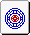and readying for a.

4. HANGING (danki [単騎]) -- the tile completed the pair.

5. DOUBLE-PON (shanpon) -- the hand had two pairs, and the tile made one of them a tri. (AKA "None of the above.")

Note that a hand may be readying to win in more than one way. For instance, a hand may have three melds and the tiles 5557 in one suit. Then it is a central win if the winning tile is a 6, but a hanging win if the winning tile is a 7.

The readying score is calculated like this:

• 0 points - two-sided
• 2 points - central
• 2 points - edge
• 2 points - hanging
• 0 points - double-tri

A rule of thumb to remember this is to think of the 2 points only given if the ready tile was "unique". But this is misleading because of hands that are ready to win in more than one of the five ways.

### Exception: Seven Pairs (chiitoitsu [七対子])

A Seven Pairs hand gets a tally of 25 points.

### Exception: All Sequence (pinfu [平和])

An All Sequence Hand gets a tally of 30 points if won by opponent's discard, and 20 points if won by self-draw. (Note that there is no self-draw bonus.)

### Calculating the Exponential (fan [翻])

The exponential (fan [翻]) is a sum of three numbers; the MULTIPLIER (yaku [役]) count, the DORA count, and a BASE exponential (bazoro [場ゾロ]).

The dora count is based on the dora tile(s), but is only counted for closed hands. At each game, a tile near the end of the wall is turned over, called the dora tile. The successor of that tile is now a scoring tile, adding one to the exponential count for each such tile the player has. Also note these common variants:

• Although not part of Toudai-shiki, some players play with an "ura-dora" (裏ドラ) rule, which creates more dora tiles in the event of a "riichi." When a player wins with riichi, the tile underneath the dora tile is also revealed, and becomes another dora tile.

• Also not part of Toudai-shiki, but also common, is the "kan-dora" rule. When there is a quad meld, another dora tile is flipped over. (It will created another ura-dora tile if those rules are also used.)

The base exponential is always 2.

### Calculation

Start with the tally. If it is even, round it up to the nearest multiple of 10 (the only possible case where the tally is not even is when it is 25 from a Seven Pairs hand.) and multiply it by 2^exponential (two raised to the power of the exponential score). This is the HAND VALUE.

If the player was dealer, multiply the hand value by 6 (representing each other player paying twice the hand value). If the player was not dealer, multiply the hand value by 4 (representing dealer paying twice the hand value, and the other two players paying the hand value). The resulting product is called the PAYOFF, and should represent approximately what the winner should get.

If the hand was won by discard, then the discarder pays the winner the entire payoff, rounded to the nearest hundred. (If the discarder is dealer, he does not pay double.)

Otherwise, the hand was won by self-draw. Then each player pays the winner the hand value, rounded up to the nearest hundred. (The hand value is doubled if either the winner or the payer is dealer.)

Note that the winner should receive a total amount close to that of the payoff.

### Limits

If the hand value is greater than 2000, then instead of using the "actual" hand value, the hand value is reduced to a LIMIT (mankan [満貫]) of 2000 points. (This is reached at about 3 exponentials for tallys greater than 70, about 4 exponentials for tallys greater than 40, and 5 exponentials for smaller tallys.) (Some books will refer to the limit as 8000 or 12000 points. They're talking about the PAYOFF limit.)

The only way to get more than the limit as a hand value is to have a lot of exponentials. The higher values are:

• LIMIT-AND-A-HALF (haneman [ハネ満]): 3000 points. 8 or 9 exponentials.

• DOUBLE LIMIT (baiman [倍満]): 4000 points. 10, 11, or 12 exponentials.

• TRIPLE LIMIT (sanbaiman [３倍満]): 6000 points.13 exponentials or more.

• QUADRUPLE LIMIT (yakuman [役満]): 8000 points. Special hands.

• SEXTUPLE LIMIT: 12000 points. Really special hands. Not possible in Toudai-shiki, but counted by some variants.

• OCTUPLE LIMIT (daburu yakuman): 16000 points. Some players use this instead of sextuple limits.

Play Mahjiangg Now!